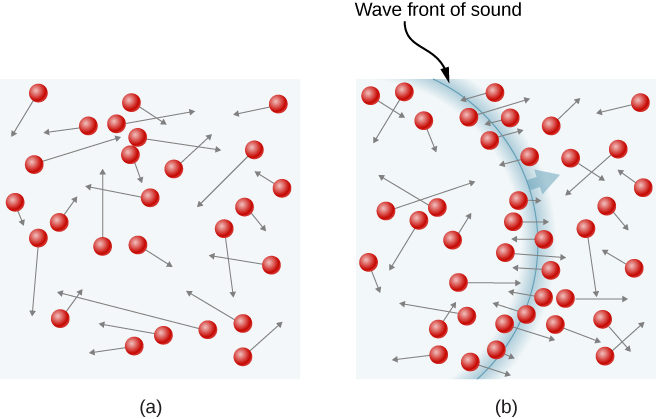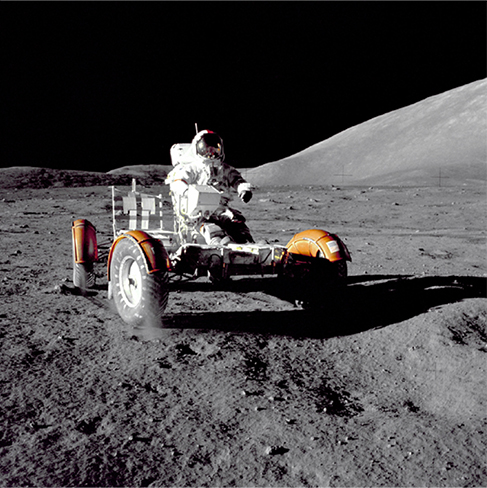# 2.2 Pressure, temperature, and rms speed  (Page 5/18)

 Page 5 / 18(a) In an ordinary gas, so many molecules move so fast that they collide billions of times every second. (b) Individual molecules do not move very far in a small amount of time, but disturbances like sound waves are transmitted at speeds related to the molecular speeds.

## Calculating temperature: escape velocity of helium atoms

To escape Earth’s gravity, an object near the top of the atmosphere (at an altitude of 100 km) must travel away from Earth at 11.1 km/s. This speed is called the escape velocity . At what temperature would helium atoms have an rms speed equal to the escape velocity?

## Strategy

Identify the knowns and unknowns and determine which equations to use to solve the problem.

## Solution

1. Identify the knowns: v is the escape velocity, 11.1 km/s.
2. Identify the unknowns: We need to solve for temperature, T . We also need to solve for the mass m of the helium atom.
3. Determine which equations are needed.
• To get the mass m of the helium atom, we can use information from the periodic table:
$m=\frac{M}{{N}_{A}}.$
• To solve for temperature T , we can rearrange
$\frac{1}{2}\phantom{\rule{0.2em}{0ex}}m\stackrel{\text{–}}{{v}^{2}}=\frac{3}{2}\phantom{\rule{0.2em}{0ex}}{k}_{\text{B}}T$

to yield
$T=\frac{m\stackrel{\text{–}}{{v}^{2}}}{3{k}_{\text{B}}}.$
4. Substitute the known values into the equations and solve for the unknowns,
$m=\frac{M}{{N}_{A}}=\frac{4.0026\phantom{\rule{0.2em}{0ex}}×\phantom{\rule{0.2em}{0ex}}{10}^{-3}\phantom{\rule{0.2em}{0ex}}\text{kg/mol}}{6.02\phantom{\rule{0.2em}{0ex}}×\phantom{\rule{0.2em}{0ex}}{10}^{23}\phantom{\rule{0.2em}{0ex}}\text{mol}}=6.65\phantom{\rule{0.2em}{0ex}}×\phantom{\rule{0.2em}{0ex}}{10}^{-27}\phantom{\rule{0.2em}{0ex}}\text{kg}$

and
$T=\frac{{\left(6.65\phantom{\rule{0.2em}{0ex}}×\phantom{\rule{0.2em}{0ex}}{10}^{-27}\phantom{\rule{0.2em}{0ex}}\text{kg}\right)\phantom{\rule{0.2em}{0ex}}\left(11.1\phantom{\rule{0.2em}{0ex}}×\phantom{\rule{0.2em}{0ex}}{10}^{3}\phantom{\rule{0.2em}{0ex}}\text{m/s}\right)}^{2}}{3\phantom{\rule{0.2em}{0ex}}\left(1.38\phantom{\rule{0.2em}{0ex}}×\phantom{\rule{0.2em}{0ex}}{10}^{-23}\phantom{\rule{0.2em}{0ex}}\text{J/K}\right)}=1.98\phantom{\rule{0.2em}{0ex}}×\phantom{\rule{0.2em}{0ex}}{10}^{4}\phantom{\rule{0.2em}{0ex}}\text{K}\text{.}$

## Significance

This temperature is much higher than atmospheric temperature, which is approximately 250 K $\left(-25\phantom{\rule{0.2em}{0ex}}\text{°}\text{C or}\phantom{\rule{0.2em}{0ex}}-10\phantom{\rule{0.2em}{0ex}}\text{°}\text{F}\right)$ at high elevation. Very few helium atoms are left in the atmosphere, but many were present when the atmosphere was formed, and more are always being created by radioactive decay (see the chapter on nuclear physics). The reason for the loss of helium atoms is that a small number of helium atoms have speeds higher than Earth’s escape velocity even at normal temperatures. The speed of a helium atom changes from one collision to the next, so that at any instant, there is a small but nonzero chance that the atom’s speed is greater than the escape velocity. The chance is high enough that over the lifetime of Earth, almost all the helium atoms that have been in the atmosphere have reached escape velocity at high altitudes and escaped from Earth’s gravitational pull. Heavier molecules, such as oxygen, nitrogen, and water, have smaller rms speeds, and so it is much less likely that any of them will have speeds greater than the escape velocity. In fact, the likelihood is so small that billions of years are required to lose significant amounts of heavier molecules from the atmosphere. [link] shows the effect of a lack of an atmosphere on the Moon. Because the gravitational pull of the Moon is much weaker, it has lost almost its entire atmosphere. The atmospheres of Earth and other bodies are compared in this chapter’s exercises.This photograph of Apollo 17 Commander Eugene Cernan driving the lunar rover on the Moon in 1972 looks as though it was taken at night with a large spotlight. In fact, the light is coming from the Sun. Because the acceleration due to gravity on the Moon is so low (about 1/6 that of Earth), the Moon’s escape velocity is much smaller. As a result, gas molecules escape very easily from the Moon, leaving it with virtually no atmosphere. Even during the daytime, the sky is black because there is no gas to scatter sunlight. (credit: Harrison H. Schmitt/NASA)

what are waves
In physics, mathematics, and related fields, a wave is a propagating dynamic disturbance (change from equilibrium) of one or more quantities
Discuss how would orient a planar surface of area A in a uniform electric field of magnitude E0 to obtain (a) the maximum flux and (b) the minimum flux through the area.
I'm just doing the first 3 with this message. but thankyou for the time your obviously intending to support us with. viva la accumulation
Marcel
Find the net capacitance of the combination of series and parallel capacitors shown belo
what is ohm?
calculate ideal gas pressure of 0.300mol,v=2L T=40°c
what is principle of superposition
what are questions that are likely to come out during exam
what is electricity
watt is electricity.
electricity ka full definition with formula
Jyoti
If a point charge is released from rest in a uniform electric field will it follow a field line? Will it do so if the electric field is not uniform?
Maxwell's stress tensor is
Yes
doris
neither vector nor scalar
Anil
if 6.0×10^13 electrons are placed on a metal sphere of charge 9.0micro Coulombs, what is the net charge on the sphere
18.51micro Coulombs
ASHOK
Is it possible to find the magnetic field of a circular loop at the centre by using ampere's law?
Is it possible to find the magnetic field of a circular loop at it's centre?
yes
Brother
The density of a gas of relative molecular mass 28 at a certain temperature is 0.90 K kgmcube.The root mean square speed of the gas molecules at that temperature is 602ms.Assuming that the rate of diffusion of a gas in inversely proportional to the square root of its density,calculate the density of
A hot liquid at 80degree Celsius is added to 600g of the same liquid originally at 10 degree Celsius. when the mixture reaches 30 degree Celsius, what will be the total mass of the liquid?
Under which topic
doris
what is electrostatics
Study of charges which are at rest
himanshu

#### Get Jobilize Job Search Mobile App in your pocket Now!By Anonymous UserBy Yacoub JayoghliBy Madison ChristianBy Ryan LoweBy Madison ChristianBy OpenStaxBy Abby SharpBy OpenStaxBy OpenStaxBy OpenStax